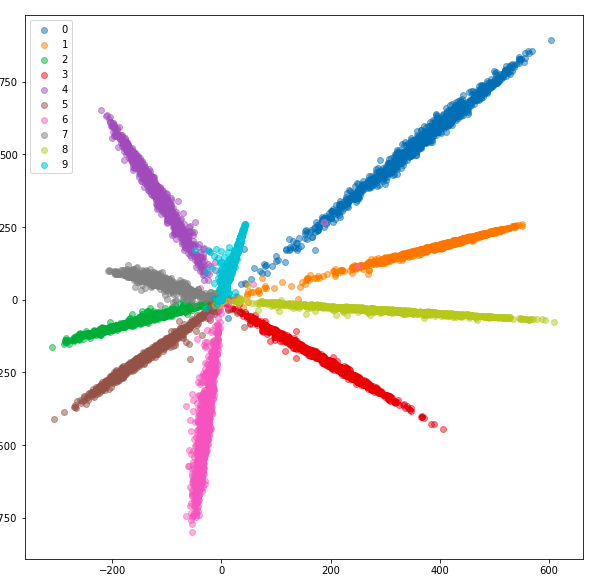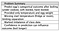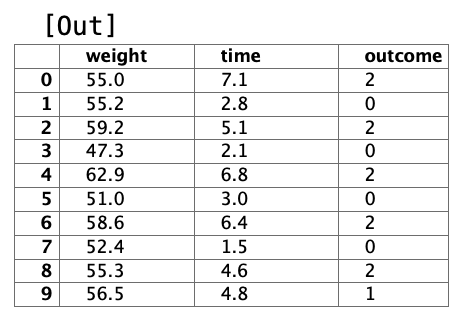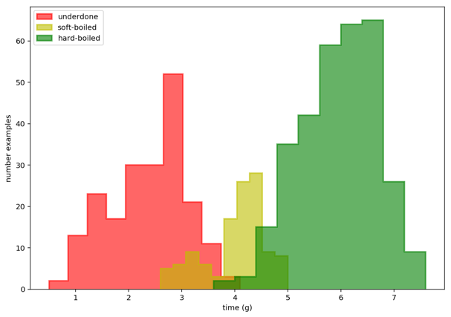1598336340

# Neural Networks Intuitions: 9. Distance Metric Learning

Welcome back to my series _Neural Networks Intuitions. _In this ninth segment, we will be looking into deep distance metric learning, the motivation behind using it, wide range of methods proposed and its applications.

Note: All techniques discussed in this article comes under Deep Metric Learning (DML) i.e distance metric learning using neural networks.

## Distance Metric Learning:

Distance Metric Learning means learning a distance in a low dimensional space which is consistent with the notion of semantic similarity. (as given in [No Fuss Distance Metric Learning using Proxies])

What does the above statement mean w.r.t image domain?

It means learning a distance in a low dimensional space(non-input space) such that similar images in the input space result in similar representation(low distance) and dissimilar images result in varied representation(high distance).

Okay, this sounds exactly what a classifier does. Isn’t it? Yes.

So how is this different from supervised image classification? Why different terminology?

Metric learning addresses the problem of open-set setup in machine learning i.e generalize to new examples at test time.

This is not possible by a feature-extractor followed by fully connected layer Classification network.

Why?

This is a very important question. The answer is as follows:

1. A classifier learns**class-specific features and not necessarily generic features.**
2. A classifier with a standard cross entropy loss maximizes inter-class distances such that the features before FC layer are linearly separable.

#metric-learning #deep-learning #siamese-networks #triplet-loss #representation-learning #deep learning

## Buddha Community1598336340

## Neural Networks Intuitions: 9. Distance Metric Learning

Welcome back to my series _Neural Networks Intuitions. _In this ninth segment, we will be looking into deep distance metric learning, the motivation behind using it, wide range of methods proposed and its applications.

Note: All techniques discussed in this article comes under Deep Metric Learning (DML) i.e distance metric learning using neural networks.

## Distance Metric Learning:

Distance Metric Learning means learning a distance in a low dimensional space which is consistent with the notion of semantic similarity. (as given in [No Fuss Distance Metric Learning using Proxies])

What does the above statement mean w.r.t image domain?

It means learning a distance in a low dimensional space(non-input space) such that similar images in the input space result in similar representation(low distance) and dissimilar images result in varied representation(high distance).

Okay, this sounds exactly what a classifier does. Isn’t it? Yes.

So how is this different from supervised image classification? Why different terminology?

Metric learning addresses the problem of open-set setup in machine learning i.e generalize to new examples at test time.

This is not possible by a feature-extractor followed by fully connected layer Classification network.

Why?

This is a very important question. The answer is as follows:

1. A classifier learns**class-specific features and not necessarily generic features.**
2. A classifier with a standard cross entropy loss maximizes inter-class distances such that the features before FC layer are linearly separable.

#metric-learning #deep-learning #siamese-networks #triplet-loss #representation-learning #deep learning1596720780

## Bayesian Neural Networks: 2 Fully Connected in TensorFlow and Pytorch

This chapter continues the series on Bayesian deep learning. In the chapter we’ll explore alternative solutions to conventional dense neural networks. These alternatives will invoke probability distributions over each weight in the neural network resulting in a single model that effectively contains an infinite ensemble of neural networks trained on the same data. We’ll use this knowledge to solve an important problem of our age: how long to boil an egg.

## Chapter Objectives:

• Become familiar with variational inference with dense Bayesian models
• Learn how to convert a normal fully connected (dense) neural network to a Bayesian neural network
• Appreciate the advantages and shortcomings of the current implementation

The data is from an experiment in egg boiling. The boil durations are provided along with the egg’s weight in grams and the finding on cutting it open. Findings are categorised into one of three classes: under cooked, soft-boiled and hard-boiled. We want the egg’s outcome from its weight and boiling time. The problem is insanely simple, so much so that the data is near being linearly separable¹⁠. But not quite, as the egg’s pre-boil life (fridge temperature or cupboard storage at room temperature) aren’t provided and as you’ll see this swings cooking times. Without the missing data we can’t be certain what we’ll find when opening an egg up. Knowing how certain we are we can influence the outcome here as we can with most problems. In this case if relatively confident an egg’s undercooked we’ll cook it more before cracking it open.Let’s have a look at the data first to see what we’re dealing with. If you want to feel the difference for yourself you can get the data at github.com/DoctorLoop/BayesianDeepLearning/blob/master/egg_times.csv. You’ll need Pandas and Matplotlib for exploring the data. (pip install — upgrade pandas matplotlib) Download the dataset to the same directory you’re working from. From a Jupyter notebook type pwd on its own in a cell to find out where that directory is if unsure.Figure 2.01 Scatter plot of egg outcomes

And let’s see it now as a histogram.Figure 2.02 Histogram of egg times by outcome

It seems I wasn’t so good at getting my eggs soft-boiled as I like them so we see a fairly large class imbalance with twice as many underdone instances and three times as many hardboiled instances relative to the soft-boiled lovelies. This class imbalance can spell trouble for conventional neural networks causing them to underperform and an imbalanced class size is a common finding.

Note that we’re not setting density to True (False is the default so doesn’t need to be specified) as we’re interested in comparing actual numbers. While if we were comparing probabilities sampled from one of the three random variables, we’d want to set density=True to normalise the histogram summing the data to 1.0.

#editors-pick #bayesian-machine-learning #deep-learning #bayesian-neural-network #neural-networks #deep learning1623135499

## No Code introduction to Neural Networks

### The simple architecture explained

Neural networks have been around for a long time, being developed in the 1960s as a way to simulate neural activity for the development of artificial intelligence systems. However, since then they have developed into a useful analytical tool often used in replace of, or in conjunction with, standard statistical models such as regression or classification as they can be used to predict or more a specific output. The main difference, and advantage, in this regard is that neural networks make no initial assumptions as to the form of the relationship or distribution that underlies the data, meaning they can be more flexible and capture non-standard and non-linear relationships between input and output variables, making them incredibly valuable in todays data rich environment.

In this sense, their use has took over the past decade or so, with the fall in costs and increase in ability of general computing power, the rise of large datasets allowing these models to be trained, and the development of frameworks such as TensforFlow and Keras that have allowed people with sufficient hardware (in some cases this is no longer even an requirement through cloud computing), the correct data and an understanding of a given coding language to implement them. This article therefore seeks to be provide a no code introduction to their architecture and how they work so that their implementation and benefits can be better understood.

Firstly, the way these models work is that there is an input layer, one or more hidden layers and an output layer, each of which are connected by layers of synaptic weights¹. The input layer (X) is used to take in scaled values of the input, usually within a standardised range of 0–1. The hidden layers (Z) are then used to define the relationship between the input and output using weights and activation functions. The output layer (Y) then transforms the results from the hidden layers into the predicted values, often also scaled to be within 0–1. The synaptic weights (W) connecting these layers are used in model training to determine the weights assigned to each input and prediction in order to get the best model fit. Visually, this is represented as:

#machine-learning #python #neural-networks #tensorflow #neural-network-algorithm #no code introduction to neural networks1621440840

## Ultimate Guide for Deep Learning with Neural Network in 2021

In deep learning with Keras, you don’t have to code a lot, but there are a few steps on which you need to step over slowly so that in the near future, you can create your models. The flow of modelling is to load data, define the Keras model, compile the Keras model, fit the Keras model, evaluate it, tie everything together, and make the predictions out of it.

But at times, you might find it confusing because of not having a good hold on the fundamentals of deep learning. Before starting your new deep learning with Keras project, make sure to go through this ultimate guide which will help you in revising the fundamentals of deep learning with Keras.

In the field of Artificial Intelligence, deep learning has become a buzzword which always finds its way in various conversations. When it comes to imparting intelligence to the machines, it has been since many years that we used Machine Learning (ML).

But, considering the current period, due to its supremacy in predictions, deep learning with Keras has become more liked and famous as compared to the old and traditional ML techniques.

### Deep Learning

Machine learning has a subset in which the Artificial Neural Networks (ANN) is trained with a large amount of data. This subset is nothing but deep learning. Since a deep learning algorithm learns from experience, it performs the task repeatedly; every time it tweaks it a little intending to improve the outcome.

It is termed as ‘deep learning’ because the neural networks have many deep layers which enables learning. Deep learning can solve any problem in which thinking is required to figure out the problem.

### **Keras **

There are many APIs, frameworks, and libraries available to get started with deep learning. But here’s why deep learning with Keras is beneficial. Keras is a high-level neural network application programming interface (API) which runs on the top of TensorFlow – which is an end-to-end machine learning platform and is an open-source. Not just Tensorflow, but also CNTK, Theano, PlaidML, etc.

It helps in commoditizing artificial intelligence (AI) and deep learning. The coding in Keras is portable, it means that using Keras you can implement a neural network while using Theano as a backend and then subsequently run it on Tensorflow by specifying the backend. Also further, it is not mandatory rather, not needed at all to change the code.

If you are wondering why deep learning is an important term in Artificial Intelligence or if you are lagging motivation to start learning deep learning with Keras, this google trends snap shows how people’s interest in deep learning has been growing steadily worldwide for the last few years.

#deep learning #deep learning with neural network #neural network1626106680

## Neural networks forward propagation deep dive 102

Forward propagation is an important part of neural networks. Its not as hard as it sounds ;-)

This is part 2 in my series on neural networks. You are welcome to start at part 1 or skip to part 5 if you just want the code.

So, to perform gradient descent or cost optimisation, we need to write a cost function which performs:

In figure 1, we can see our network diagram with much of the details removed. We will focus on one unit in level 2 and one unit in level 3. This understanding can then be copied to all units. (ps. one unit is one of the circles below)

Our goal in forward prop is to calculate A1, Z2, A2, Z3 & A3

Just so we can visualise the X features, see figure 2 and for some more info on the data, see part 1.

## Initial weights (thetas)

As it turns out, this is quite an important topic for gradient descent. If you have not dealt with gradient descent, then check this article first. We can see above that we need 2 sets of weights. (signified by ø). We often still calls these weights theta and they mean the same thing.

We need one set of thetas for level 2 and a 2nd set for level 3. Each theta is a matrix and is size(L) * size(L-1). Thus for above:

• Theta1 = 6x4 matrix

• Theta2 = 7x7 matrix

We have to now guess at which initial thetas should be our starting point. Here, epsilon comes to the rescue and below is the matlab code to easily generate some random small numbers for our initial weights.

``````function weights = initializeWeights(inSize, outSize)
epsilon = 0.12;
weights = rand(outSize, 1 + inSize) * 2 * epsilon - epsilon;
end
``````

After running above function with our sizes for each theta as mentioned above, we will get some good small random initial values as in figure 3

. For figure 1 above, the weights we mention would refer to rows 1 in below matrix’s.

Now, that we have our initial weights, we can go ahead and run gradient descent. However, this needs a cost function to help calculate the cost and gradients as it goes along. Before we can calculate the costs, we need to perform forward propagation to calculate our A1, Z2, A2, Z3 and A3 as per figure 1.

#machine-learning #machine-intelligence #neural-network-algorithm #neural-networks #networks